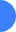Multidimensional autoregressionNext: Time domain versus frequency Up: Reproducible Documents

# Multidimensional autoregression

Jon Claerbout

Occam's razor says we should try understand the world by the simplest explanation. So, how do we decompose a complicated thing into its essential parts? That's far too difficult a question, but the word ``covariance'' points the way. If things are found statistically connected (covary), the many might be explainable by a few. For example a one-dimensional waveform can excite a wave equation filling a 3-D space. The values in that space will have a lot of covariance. In this chapter we take multidimensional spaces full of numbers and answer the question, ``what causal differential (difference) equation might have created these numbers?'' Our answer here, an autoregressive filter, does the job imperfectly, but it is a big step away from complete ignorance. As the book progresses we find three kinds of uses: (1) filling in missing data and uncontrolled parts of models, (2) preparing residuals for data fitting, (3) providing ``prior'' models for preconditioning an estimation.

Recall that residuals (and preconditioning variables) should be Independent, and Identically Distributed (IID). In practice the ``ID'' means all residuals should have the same variance, and the preceding ``I'' means likewise in Fourier space (whiteness). This is the ``I'' chapter. Conceptually we might jump in and out of Fourier space, but here we learn processes in physical space that whiten in Fourier space. In earlier chapters we transformed from a physical space to something more like an IID space when we said, ``Topography is smooth, so let us estimate and view instead its derivative.''

The branch of mathematics introduced here is young. Physicists seem to know nothing of it, perhaps because it begins with time not being a continuous variable. About 100 years ago people looked at market prices and wondered why they varied from day to day. To try to make money from the market fluctuations they schemed to try to predict prices. That is a good place to begin. The subject is known as ``time-series analysis.'' In this chapter we define the autoregression filter, also known as the prediction-error filter (PEF). It gathers statistics for us. It gathers not the autocorrelation or the spectrum directly but it gathers them indirectly as the inverse of the amplitude spectrum of its input. Although time-series analysis is a one dimensional study, we naturally use the helix to broaden it to multidimensional space. The PEF leads us to the ``inverse-covariance matrix'' of statistical estimation theory. Theoreticians tell us we need this before we can properly find a solution. Here we see how to go after it.Multidimensional autoregressionNext: Time domain versus frequency Up: Reproducible Documents

2013-07-26